## A quick review of World’s Inequality: Income and Education

Publicado: 17 julio 2022 a las 2:00 pm

Categorías: Artículos

Por Santiago Chavez

##### What is the HDI and what does it tell us?

The Human Development Index (HDI) is a report created by the United Nations. The objective is to measure key dimensions of human development as follows: a long and healthy life, being knowledgeable and have a decent standard of living.

How does the United Nations measure this?

• The health dimension is assessed by life expectancy at birth.
• The education dimension is measured by mean of years of schooling for adults aged 25 years and more and expected years of schooling for children of school entering age.
• The standard of living dimension is measured by gross national income per capita.

The report is part of the Millennium Development Goals to eradicate the extreme poverty and hunger in the world. The 2019 HDI covers 189 countries. This index can be used by all the countries to evaluate the national policy decisions and to track the improvements in several subjects related to human development.Then, the visualization of this index can be very useful to question what should be the government policy priorities.

The example below uses Coefficient of Human Inequality Index, Income Inequality Index and Education Inequality Index. The aim of the following data visualization is to explore the relation between income and education in the Americas region.

First, we import a map and plot the regions:

``````#Import a map and plot regions
globeMap <- read.csv2("~/Personal/Maestria Ingenieria Industrial/Semestre 2/Inteligencia de Negocios/Prof Juan Salamanca - Visualizacion/Proyecto Final/map_2022.csv", header=TRUE, stringsAsFactors=TRUE)

#Add latitude and longitude to the original dataset
library(ggplot2)

ggplot(globeMap, aes(x = long, y = lat, group = group)) +
geom_polygon(aes(fill=`sub.region`))+
scale_fill_manual(values =  c("#c8522c","#4bafd0","#d34459","#64b948","#a35ac7","#b4b335","#6a75c8","#d24699","#5dc18a","#a04a6d","#598233","#d389c3","#388864","#d07a6c","#b5a861","#886a2c","#db923b"))+
labs(title = "Regions of the World", caption = "Empty territories have no index. Source Uknown", x = "Longitude", y= "Latitude")+ theme_bw()``````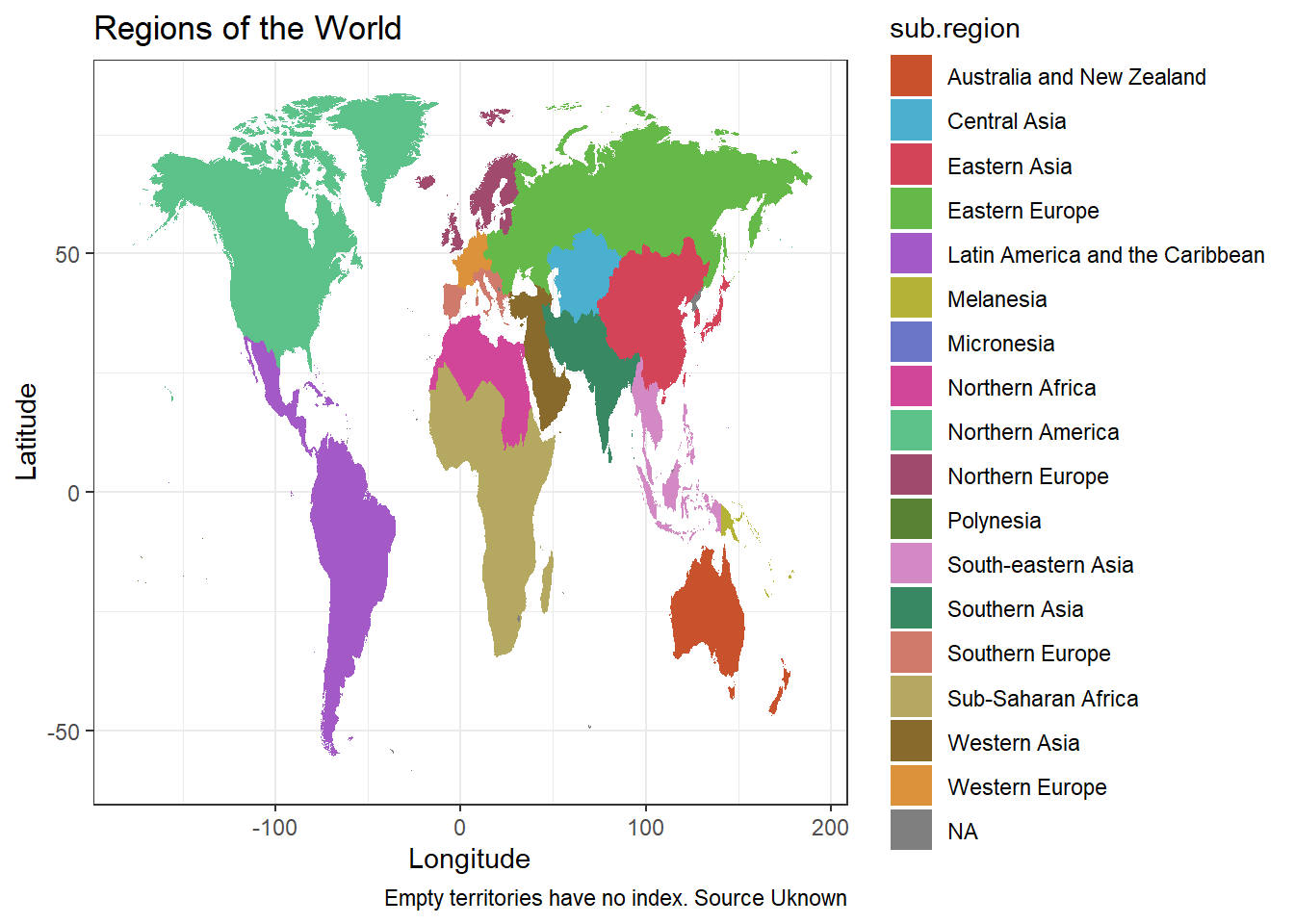##### A broad level dataset visualization

We begin to visualize the dataset at a broad level. In this case we are using the Coefficient of Human Inequality dataset. A quick exploration of the dataset shows that it has 1670 observations, 6 variables and collect data from 10 years (2010-2019). This forms a matrix of 1670 rows and 6 columns.

``````#Import Dataset
IHDI_Coefficient <- read.csv("~/Personal/Maestria Ingenieria Industrial/Semestre 2/Inteligencia de Negocios/Prof Juan Salamanca - Visualizacion/Proyecto Final/Coefficient_of_human_inequality_2010-2019.csv", sep=";", stringsAsFactors=TRUE)

#Get primary data description
str(IHDI_Coefficient)``````
``````## 'data.frame':    1670 obs. of  6 variables:
##  \$ X       : int  1 2 3 4 5 6 7 8 9 10 ...
##  \$ HDI.Rank: int  169 69 91 148 46 81 8 18 88 58 ...
##  \$ Country : Factor w/ 167 levels "Afghanistan",..: 1 2 3 4 5 6 7 8 9 10 ...
##  \$ ISO3    : Factor w/ 167 levels "AFG","AGO","ALB",..: 1 3 43 2 4 5 6 7 8 15 ...
##  \$ variable: int  2010 2010 2010 2010 2010 2010 2010 2010 2010 2010 ...
##  \$ value   : Factor w/ 351 levels "10","10,1","10,2",..: NA 28 NA 275 91 10 329 325 35 41 ...``````

Since the variable value is factor, we will have to switch it into a numerical variable.

``````#Convert from factor to numerical
IHDI_Coefficient\$value = as.numeric(IHDI_Coefficient\$value)

#Get primary data description
summary(IHDI_Coefficient\$value)``````
``````##    Min. 1st Qu.  Median    Mean 3rd Qu.    Max.    NA's
##     1.0    77.0   172.0   173.6   268.0   351.0     216``````
``levels(as.factor(IHDI_Coefficient\$variable))``
``##   "2010" "2011" "2012" "2013" "2014" "2015" "2016" "2017" "2018" "2019"``

This is the distribution of the Coefficient Inequality Index across 10 years. The lowest inequality is 1 and the highest is 351. The median is 172, which means that half of the dataset has an Coefficient Inequality Index above 172. Let’s create a chart to visualize it better.

``````library(ggplot2)
overallDist <- ggplot(IHDI_Coefficient,aes(x=value))
overallDist <- overallDist + geom_density(alpha=.2, fill="gold")
overallDist <- overallDist + geom_vline (aes ( xintercept = 172, color = 'black'))
overallDist <- overallDist + geom_text (x=172, y=0.015, label="Global median")
overallDist <- overallDist + labs(title = "Coefficient of Human Inequality (IHDI)", subtitle = "Aggregated from 2010-2019", x = "IHDI Index") + xlim(c(0,351))
overallDist <- overallDist + theme(legend.position = "none")
overallDist``````
``## Warning: Removed 216 rows containing non-finite values (stat_bin).``
``## Warning: Removed 216 rows containing non-finite values (stat_density).``
``## Warning: Removed 2 rows containing missing values (geom_bar).``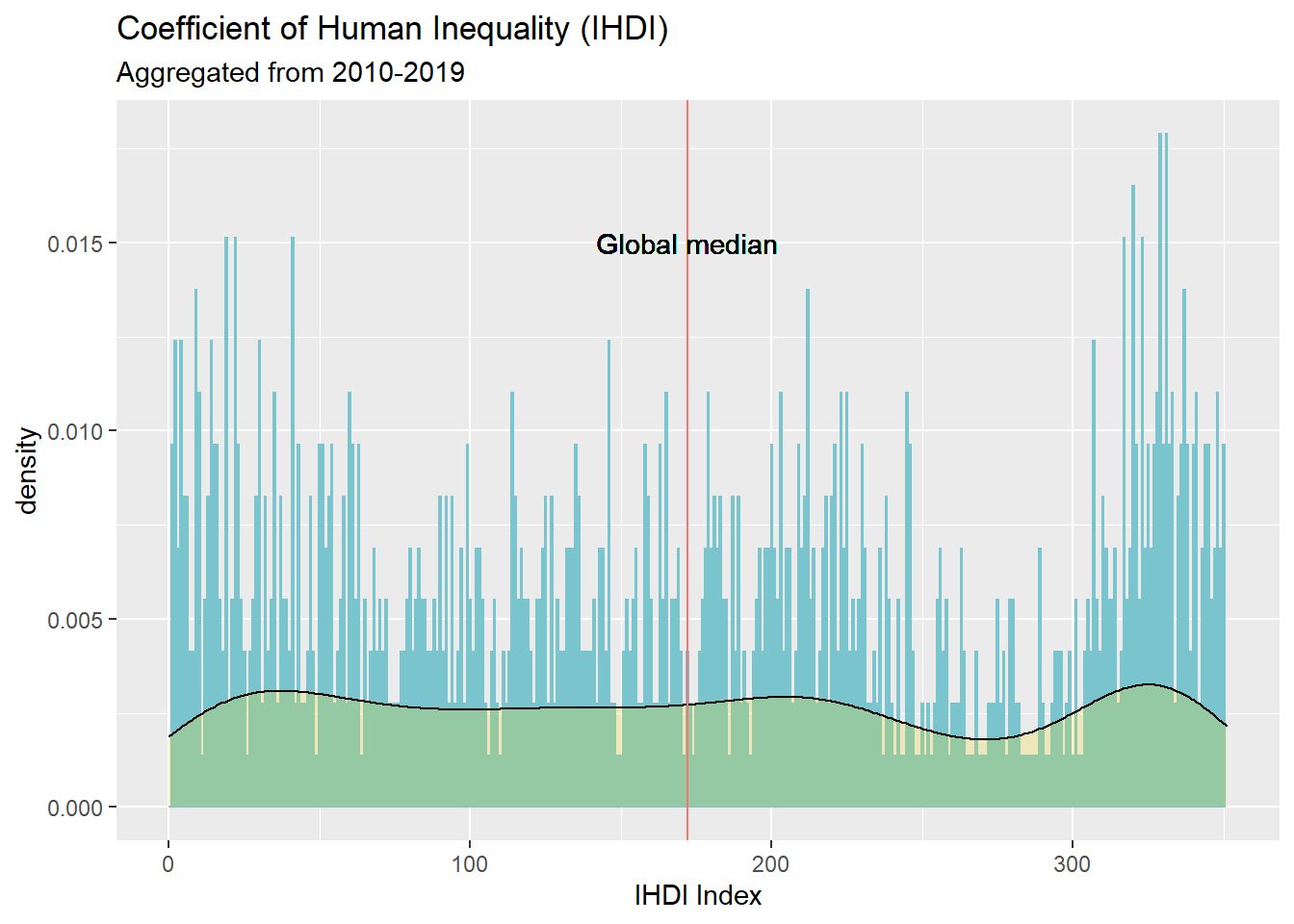IHDI adjusts the Human Development Index (HDI) for inequality in the distribution of each dimension across the population. At a global scale, the half of the world is in acceptable condition. Let’s keep digging in the data.

Now we will present the Income Inequality Index. Just in case!

A quick exploration of the dataset shows that it has 1700 observations, 6 variables and collect data from 10 years (2010-2019). This forms a matrix of 1700 rows and 6 columns.

``````#Import Dataset
IHDI_Income <- read.csv("~/Personal/Maestria Ingenieria Industrial/Semestre 2/Inteligencia de Negocios/Prof Juan Salamanca - Visualizacion/Proyecto Final/Inequality_Income_2010-2019.csv", sep=";", stringsAsFactors=TRUE)

# Get primary data description
str(IHDI_Income)``````
``````## 'data.frame':    1700 obs. of  6 variables:
##  \$ X       : int  1 2 3 4 5 6 7 8 9 10 ...
##  \$ HDI.Rank: int  169 69 91 148 46 81 8 18 88 58 ...
##  \$ Country : Factor w/ 170 levels "Afghanistan",..: 1 2 3 4 5 6 7 8 9 10 ...
##  \$ ISO3    : Factor w/ 170 levels "AFG","AGO","ALB",..: 1 3 43 2 4 5 6 7 8 15 ...
##  \$ variable: int  2010 2010 2010 2010 2010 2010 2010 2010 2010 2010 ...
##  \$ value   : Factor w/ 311 levels "10,2","10,3",..: NA 40 NA 236 220 6 60 47 257 136 ...``````

Since the variable value is factor, we will have to switch it into a numerical variable

``````#Convert from factor to numerical
IHDI_Income\$value = as.numeric(IHDI_Income\$value)

#Get primary data description
summary(IHDI_Coefficient\$value)``````
``````##    Min. 1st Qu.  Median    Mean 3rd Qu.    Max.    NA's
##     1.0    77.0   172.0   173.6   268.0   351.0     216``````
``levels(as.factor(IHDI_Coefficient\$variable))``
``##   "2010" "2011" "2012" "2013" "2014" "2015" "2016" "2017" "2018" "2019"``

This is the distribution of the Income Inequality Index across 10 years. The lowest inequality is 1 and the highest is 351. The median is 172, which means that half of the dataset has a Income Inequality Index above 172. Let’s visualize that in a chart.

``````library(ggplot2)
overallDist <- ggplot(IHDI_Income,aes(x=value))
overallDist <- overallDist + geom_density(alpha=.2, fill="gold")
overallDist <- overallDist + geom_vline (aes ( xintercept = 172, color = 'black'))
overallDist <- overallDist + geom_text (x=172, y=0.025, label="Global median")
overallDist <- overallDist + labs(title = "Income Inequality", subtitle = "Aggregated from 2010-2019", x = "IHDI Index") +
xlim(c(1,351))
overallDist <- overallDist + theme(legend.position = "none")
overallDist``````
``## Warning: Removed 211 rows containing non-finite values (stat_bin).``
``## Warning: Removed 211 rows containing non-finite values (stat_density).``
``## Warning: Removed 2 rows containing missing values (geom_bar).``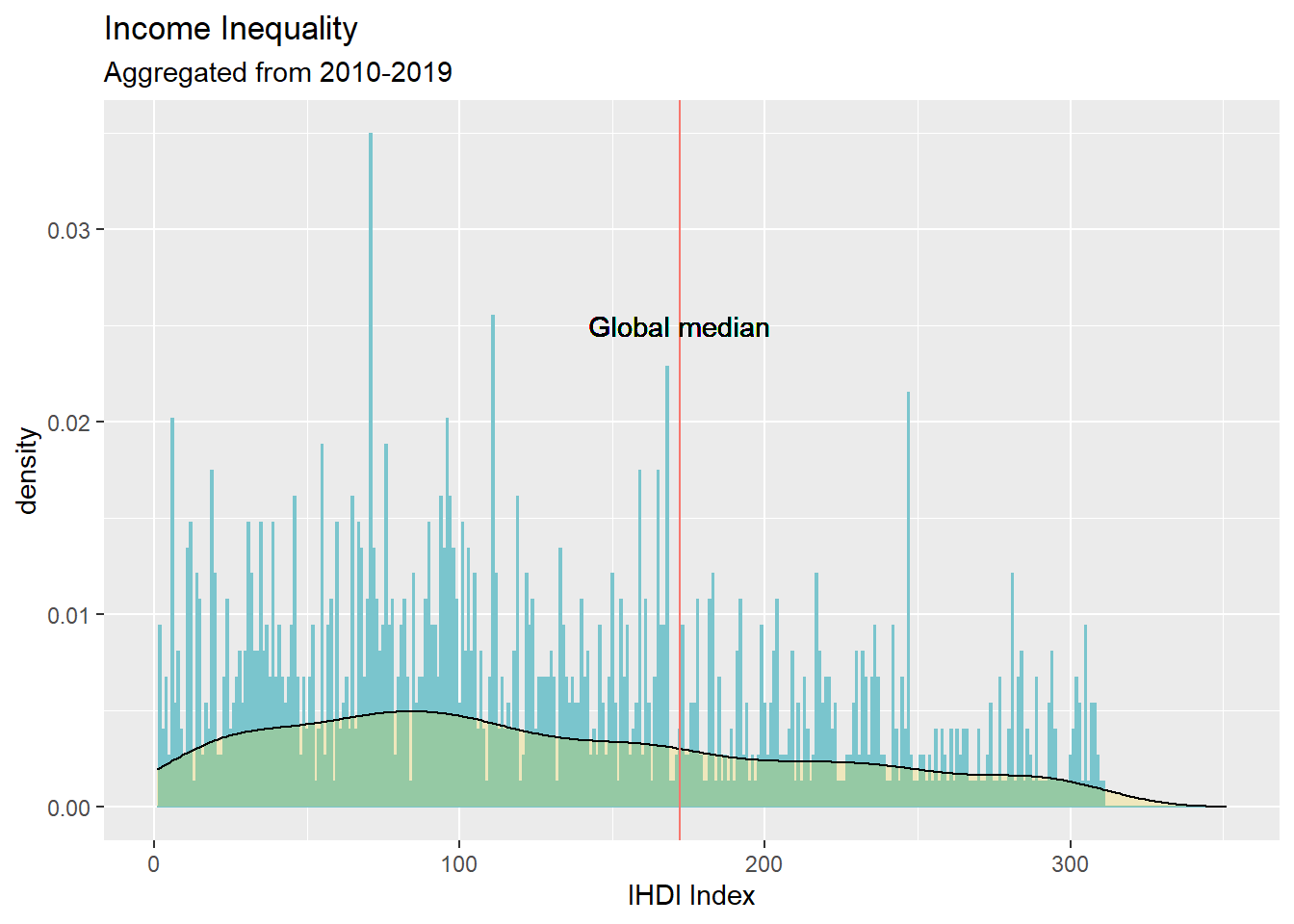Although the median looks good, we can observe that density starts to move to the left side.Let’s keep digging in the data.

Now is time to check the Education Inequality Index. A quick exploration of the dataset shows that it has 1820 observations, 6 variables and collect data from 10 years (2010-2019). This forms a matrix of 1820 rows and 6 columns.

``````#Import Dataset

IHDI_Edu <- read.csv2("~/Personal/Maestria Ingenieria Industrial/Semestre 2/Inteligencia de Negocios/Prof Juan Salamanca - Visualizacion/Proyecto Final/Inequality_Education_2010-2019.csv", stringsAsFactors=TRUE)

# Get primary data description
str(IHDI_Edu)``````
``````## 'data.frame':    1820 obs. of  6 variables:
##  \$ X       : int  1 2 3 4 5 6 7 8 9 10 ...
##  \$ HDI.Rank: int  169 69 91 36 148 46 81 8 18 88 ...
##  \$ Country : Factor w/ 182 levels "Afghanistan",..: 1 2 3 4 5 6 7 8 9 10 ...
##  \$ ISO3    : Factor w/ 182 levels "AFG","AGO","ALB",..: 1 3 47 4 2 6 7 8 9 10 ...
##  \$ variable: int  2010 2010 2010 2010 2010 2010 2010 2010 2010 2010 ...
##  \$ value   : num  39.3 12.7 NA NA 26.2 12.1 6.5 1.7 2.4 12 ...``````

This time the switch of the value variable is not required, it is already set as numeric. This is the distribution of the Education Inequality Index across 10 years. The lowest inequality is 0.7 and the highest is 50.10. The median is 19.99, which means that half of the dataset has an Education Inequality index above 19.99. Let’s visualize that in a chart.

``````# Get primary data description
summary(IHDI_Edu\$value)``````
``````##    Min. 1st Qu.  Median    Mean 3rd Qu.    Max.    NA's
##    0.70    6.30   17.10   19.99   32.20   50.10     251``````
``levels(as.factor(IHDI_Edu\$variable))``
``##   "2010" "2011" "2012" "2013" "2014" "2015" "2016" "2017" "2018" "2019"``
``````library(ggplot2)
overallDist <- ggplot(IHDI_Edu,aes(x=value))
overallDist <- overallDist + geom_density(alpha=.2, fill="gold")
overallDist <- overallDist + geom_vline (aes ( xintercept = 17.1, color = 'black'))
overallDist <- overallDist + geom_text (x=17.1, y=0.04, label="Global median")
overallDist <- overallDist + labs(title = "Education Inequality", subtitle = "Aggregated from 2010-2019", x = "IHDI Index")+
xlim(c(.7,51))
overallDist <- overallDist + theme(legend.position = "none")
overallDist``````
``## Warning: Removed 251 rows containing non-finite values (stat_bin).``
``## Warning: Removed 251 rows containing non-finite values (stat_density).``
``## Warning: Removed 2 rows containing missing values (geom_bar).``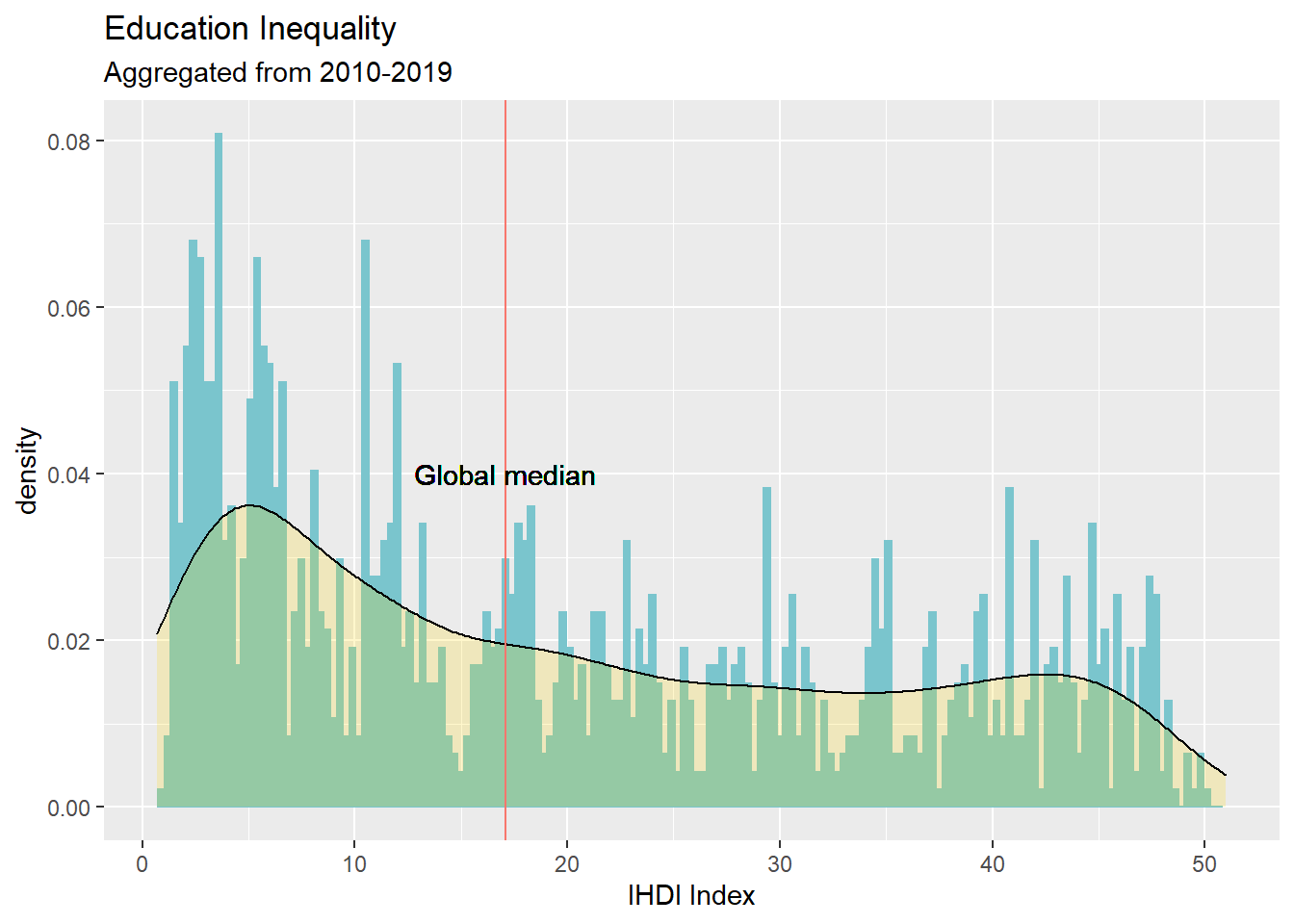The next step is to create a new dataset including regions using the dplyr library. The objective is to mark each country with its corresponding region.

``library(dplyr)``
``````##
## Attaching package: 'dplyr'``````
``````## The following objects are masked from 'package:stats':
##
##     filter, lag``````
``````## The following objects are masked from 'package:base':
##
##     intersect, setdiff, setequal, union``````
``````#Create new dataset for get regions
regions <- select(globeMap,ISO3,region)
regions <- distinct(regions)
``````##   ISO3   region
## 1  ABW Americas
## 2  AFG     Asia
## 3  AGO   Africa
## 4  AIA Americas
## 5  ALB   Europe
## 6  FIN   Europe``````
``````library(dplyr)
Edu_MainData <- left_join(IHDI_Edu,regions,by=c('ISO3' = 'ISO3'))

library(ggplot2)
overallDist <- ggplot(Edu_MainData,aes(x=value))
overallDist <- overallDist + geom_density(alpha=.2, fill="gold")
overallDist <- overallDist + geom_vline (aes ( xintercept = 21, color = 'black'))
overallDist <- overallDist + geom_text (x=19.99, y=0.3, label="Global Median")
overallDist <- overallDist + facet_grid(as.character(region)~.)
overallDist <- overallDist + labs(title = "Education Inequality", subtitle = "Aggregated from 2010-2019", x = "IHDI Index")+
xlim(c(.7,51))
overallDist <- overallDist + theme(legend.position = "none")
overallDist``````
``## Warning: Removed 251 rows containing non-finite values (stat_bin).``
``## Warning: Removed 251 rows containing non-finite values (stat_density).``
``## Warning: Removed 12 rows containing missing values (geom_bar).``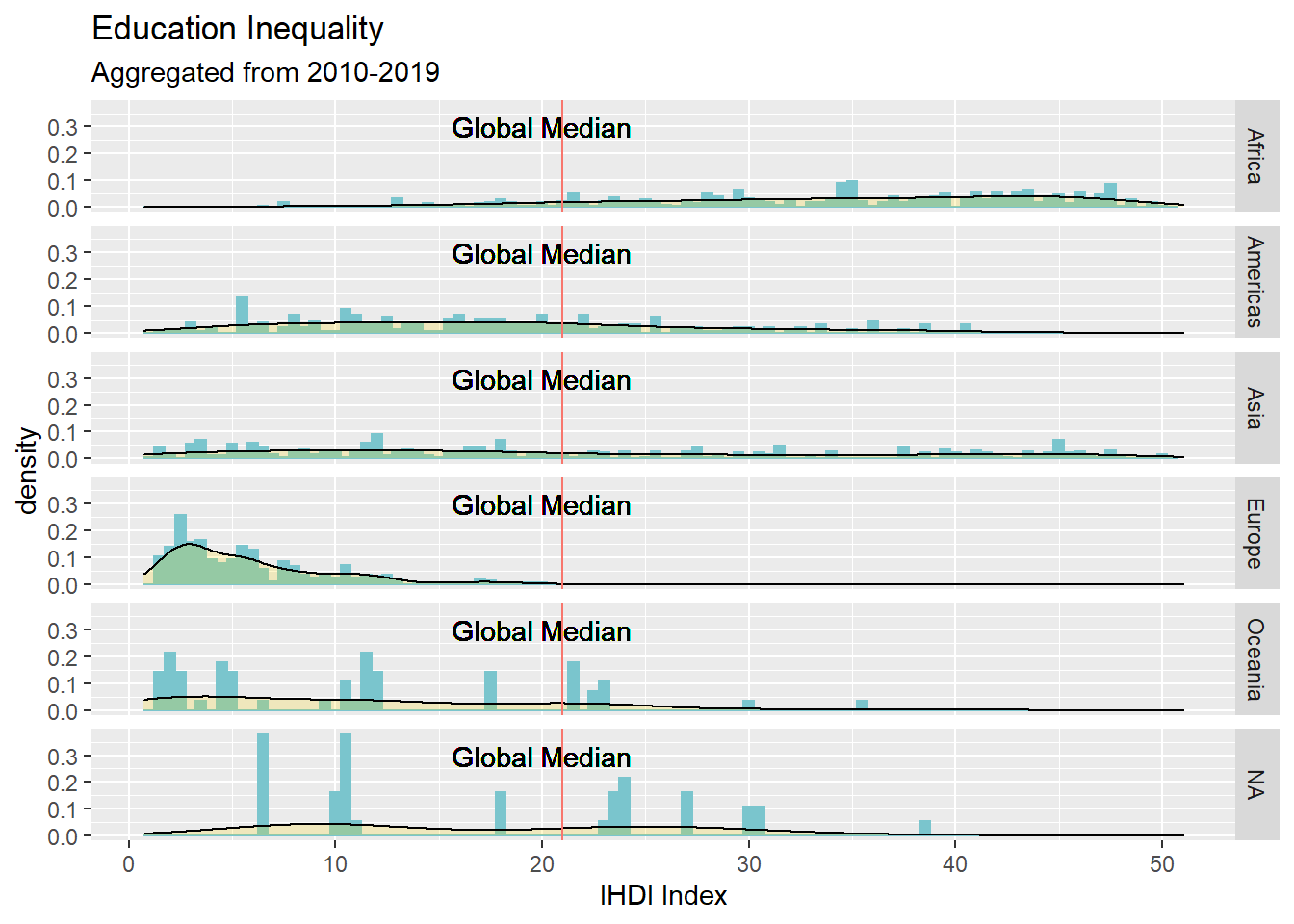We start to see differences among the continents. For example density is laid on the right side for Africa. This means the Education Inequality Index is greater, which means there is more inequality in that subject.

``````# Subset by year
Adjusted_2019 <- subset (Edu_MainData , Edu_MainData\$variable == '2019')
# Sort by value
# Save the sorted list of countries

``````library(ggplot2)
# assign dataset
plot <- plot + aes(x=sortedCountryNamesAdjusted, y=value)
# Median 185/2
plot <- plot + geom_vline(xintercept = 19.99, color = 'black')
# Median label
plot <- plot + geom_text(aes(x= 19.99, y= 47, label="Global Median", angle = 90), color = 'gray59', size=2.5)
plot <- plot + geom_bar(aes(x=sortedCountryNamesAdjusted, y=value, fill=as.factor(region)), stat = "identity", alpha= 0.8)
plot <- plot + geom_text(aes (y=value + 0.03, label=sortedCountryNamesAdjusted, angle = 90), size=1 )
plot <- plot + scale_fill_manual(values = colorSequence1)
# Customize legends
plot <- plot + guides(fill=guide_legend(title="Continents"))
# Customize labels
plot <- plot + labs(title = "Indexed education enequality in the world", subtitle = "Sorted chart. Year 2019",x = "World countries", y = "Indexed Education Inequality")
# adjust background, remove x label
plot <- plot + theme(legend.position=c(0.93,0.75), axis.text.x = element_blank())
# Hide x tick marks, labels, and grid lines
plot <- plot + scale_x_discrete(breaks=NULL)
# plot output
plot``````
``## Warning: Removed 10 rows containing missing values (position_stack).``
``## Warning: Removed 10 rows containing missing values (geom_text).``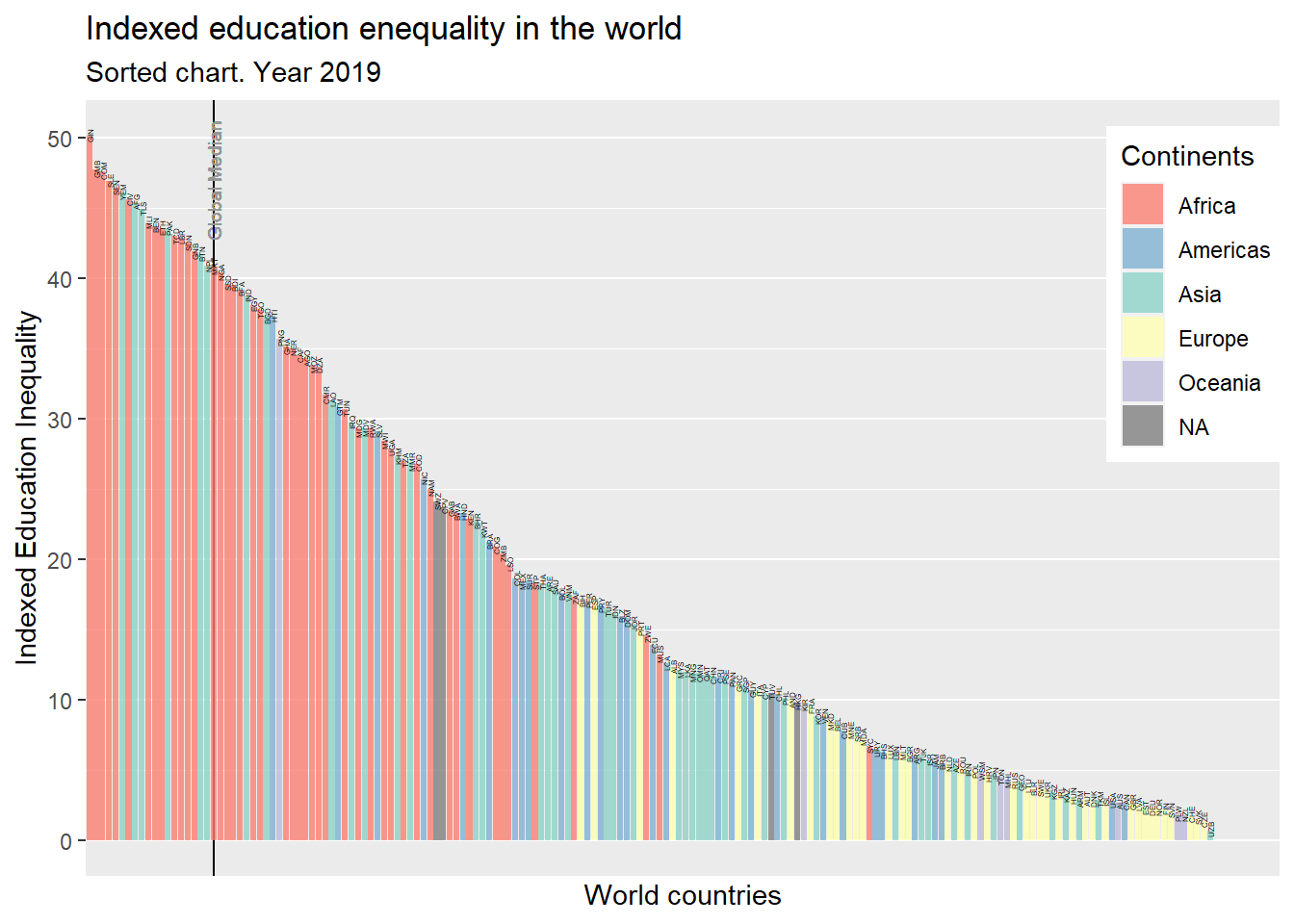By average, the top five most unequal countries in 2019 are: Guinea, Gambia, Comoros, Sierra Leona, and Senegal. All African countries. The top five most equal countries in 2019 are: Uzbekistan, Czech Republic, Slovakia, Switzerland and New Zealand. Three out of five countries ex URSS countries. Here we can confirm the fact mentioned before. In the previous graph, the density was laid on the right side for Africa.

``````library(dplyr)
#Create new dataset for get subregions
subregions <- select(globeMap,ISO3,sub.region)
subregions <- distinct(subregions)

colorSequence2 <- c("#8dd3c7","#ffffb3")

library(dplyr)
Edu_ParallelAmericas <- left_join(IHDI_Edu,subregions,by=c('ISO3' = 'ISO3'))``````

Let’s check closer the Americas region

##### A Parallel between Northern America and Latin America and the Caribbean
``````# Subset by year
ParallelAmericas_2019 <- subset (Edu_ParallelAmericas , Edu_ParallelAmericas\$variable == '2019')
# Sort by value
ParallelAmericas_2019 <- ParallelAmericas_2019[order(-ParallelAmericas_2019\$value),]
# Save the sorted list of countries
sortedCountryNamesAdjusted <- factor(ParallelAmericas_2019\$ISO3, levels = ParallelAmericas_2019\$ISO3)

# Subset Northern America and Latin America and the Caribbean
LATAM_America_2019 <- subset(ParallelAmericas_2019, (ParallelAmericas_2019\$sub.region == 'Latin America and the Caribbean' | ParallelAmericas_2019\$sub.region == 'Northern America'))
# Sort by value
LATAM_America_2019 <- LATAM_America_2019[order(-LATAM_America_2019\$value),]
# Save the sorted list of countries
sortedCountryNamesAdjusted <- factor(LATAM_America_2019\$ISO3, levels = LATAM_America_2019\$ISO3)``````
``````plot <- ggplot(LATAM_America_2019)
plot <- plot + aes(x=sortedCountryNamesAdjusted, y=value)
plot <- plot + geom_vline(xintercept = 19.99, color = 'gray59')
plot <- plot + geom_text(aes(x= 19.99, y= 18, label="Global Median", angle = 90), color = 'gray59', size=3)
plot <- plot + geom_bar(aes(x=sortedCountryNamesAdjusted, y=value, fill=as.factor(sub.region)), stat = "identity", alpha= 0.8)
plot <- plot + geom_text(aes (y=value + 0.03, label=sortedCountryNamesAdjusted, angle = 90), size=3 )
plot <- plot + scale_fill_manual(values = colorSequence2)
plot <- plot + guides(fill=guide_legend(title="Continent"))
plot <- plot + labs(title = "Indexed Education Inequality in Latin America and the Caribbean and Northern America", subtitle = "Sorted chart. Year 2019",x = "American countries", y = "Indexed Education Inequality")
plot <- plot + theme(legend.position=c(0.4,0.75), axis.text.x = element_blank())
plot <- plot + scale_x_discrete(breaks=NULL)
plot``````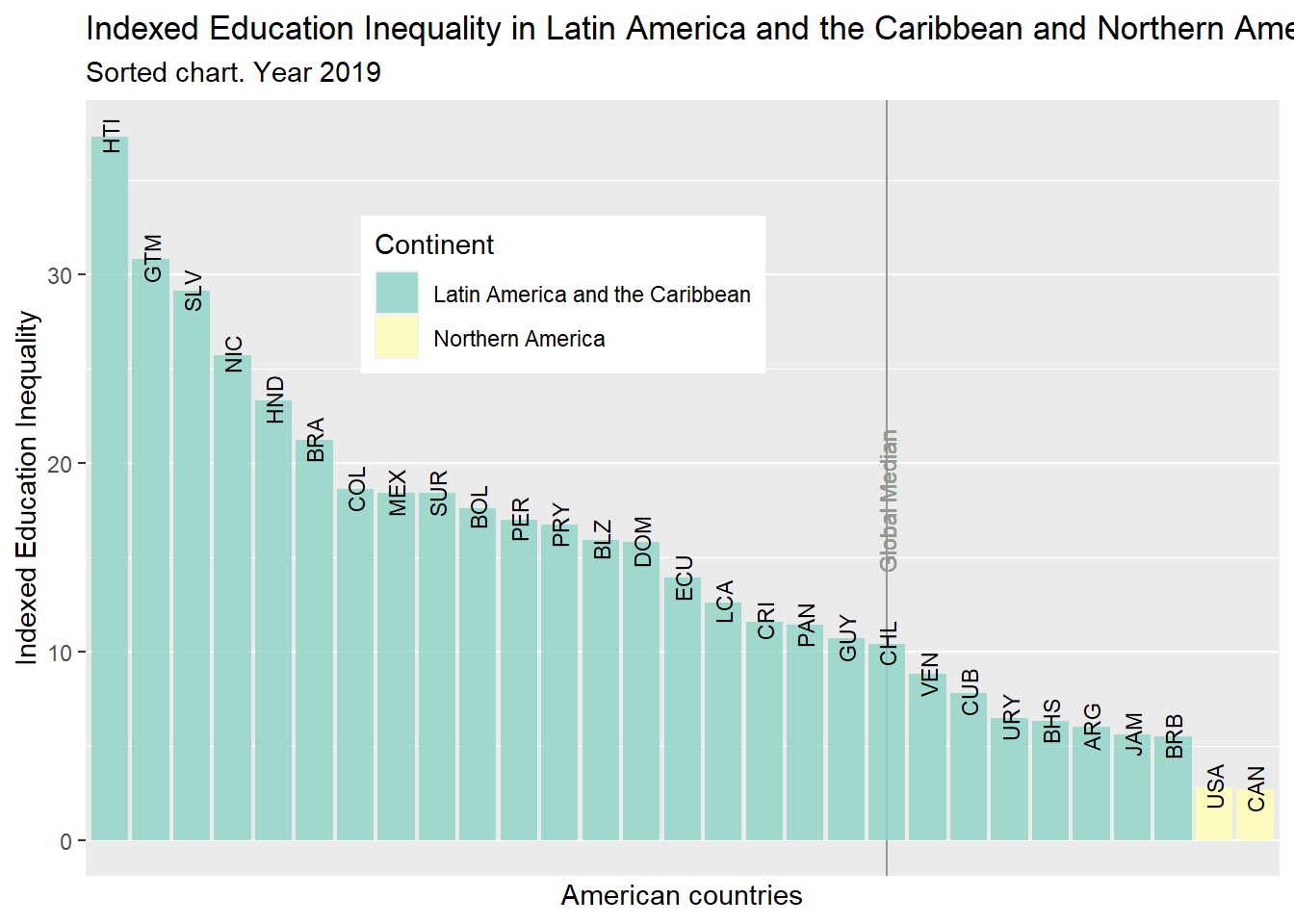Canada, United States, Barbados, Jamaica, and Argentina head the top of the list like the most equal in terms of education. In opposite Haiti, Guatemala, El Salvador, Nicaragua, Honduras, Brazil, and Colombia are in tail of the distribution and below the global median. Almost two third of the American countries are below the global median.

##### Data in the map

Finally, we will use Choroplet map to visualize the differences among American countries in terms of income and education inequality in 2019

``````library(dplyr)
Edu_MainData <- left_join(IHDI_Edu,regions,by=c('ISO3' = 'ISO3'))
Income_MainData <- left_join(IHDI_Income,regions,by=c('ISO3' = 'ISO3'))

Edu_MainData\$name <- "Education"
Income_MainData\$name <- "Income"

IHDI_Education <- subset(Edu_MainData, Edu_MainData\$region == 'Americas')
IHDI_Income <- subset(Income_MainData, Income_MainData\$region == 'Americas')

IHDIaggregated <- IHDI_Education
IHDIaggregated <- rbind(IHDIaggregated, IHDI_Income)``````
``````# Subset the main dataset 2019
IHDI_2019 <- subset(IHDIaggregated, IHDIaggregated\$variable == "2019")

# Add data to the map
mapData <- left_join(IHDI_2019,globeMap, by = c("ISO3"="ISO3"))

mapDataEducation <- subset(mapData,mapData\$name == "Education")

myMap <- ggplot(mapDataEducation, aes(x=long, y=lat, group = as.factor(group)))

myMap <- myMap + geom_polygon(aes(fill = value))
myMap <- myMap + scale_fill_viridis_c(option="plasma")
myMap <- myMap + labs(title = "Americas' inequality in Education in 2019", caption = "Source UN", x = "Longitude", y= "Latitude")
myMap <- myMap + theme_bw()
myMap``````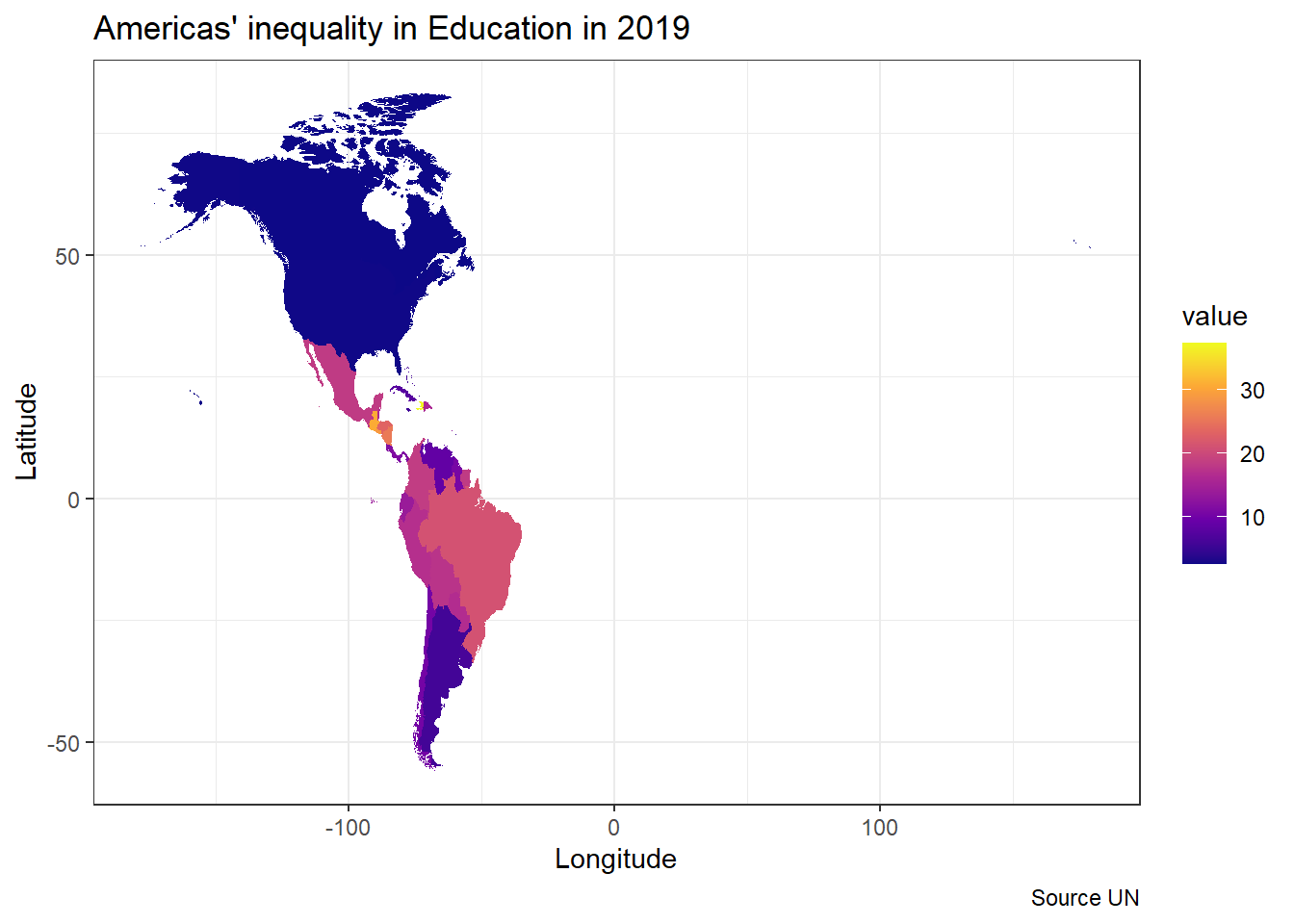``````# Subset the main dataset 2019
IHDI_2019 <- subset(IHDIaggregated, IHDIaggregated\$variable == "2019")

# Add data to the map
mapData2 <- left_join(IHDI_2019,globeMap, by = c("ISO3"="ISO3"))

mapDataIncome <- subset(mapData2,mapData2\$name == "Income")

mapIncome <- ggplot(mapDataIncome, aes(x=long, y=lat, group = as.factor(group)))
mapIncome <- mapIncome + geom_polygon(aes(fill = value))
mapIncome <- mapIncome + scale_fill_viridis_c(option="plasma")
mapIncome <- mapIncome + labs(title = "Americas' inequality in Income for 2019", caption = "Source UN", x = "Longitude", y= "Latitude")
mapIncome <- mapIncome + theme_bw()
mapIncome``````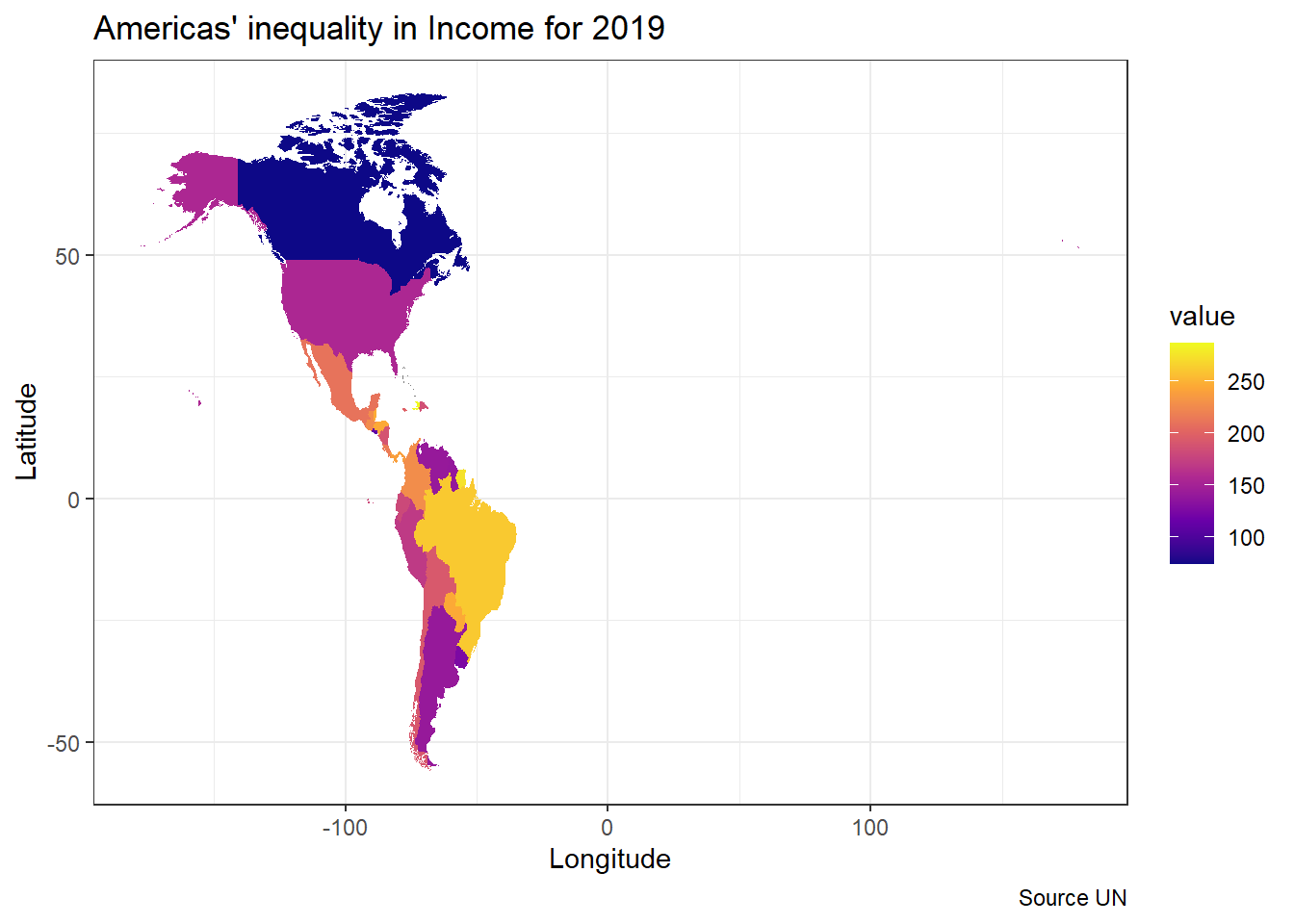#### Conclusion

In conclusion, education and income are variables very related. We observe that countries with high income are more likely to have low education inequality in his society. Africa is historically the most affected continent in terms of education.In 2019, the top five most unequal countries in 2019 were: Guinea, Gambia, Comoros, Sierra Leona, and Senegal. All African countries. The top five most equal countries in 2019 are: Uzbekistan, Czech Republic, Slovakia, Switzerland and New Zealand. Three out of five countries ex URSS countries.

Speaking about American continent, Canada, United States, Barbados, Jamaica, and Argentina head the top of the list like the most equal countries in terms of education. In opposite Haiti, Guatemala, El Salvador, Nicaragua, Honduras, Brazil, and Colombia are in tail of the distribution and below the global median. The Choroplet map show an idea: Northern American countries have better income equality than Latin American.

Source

https://rpubs.com/Sanchavaro/924603